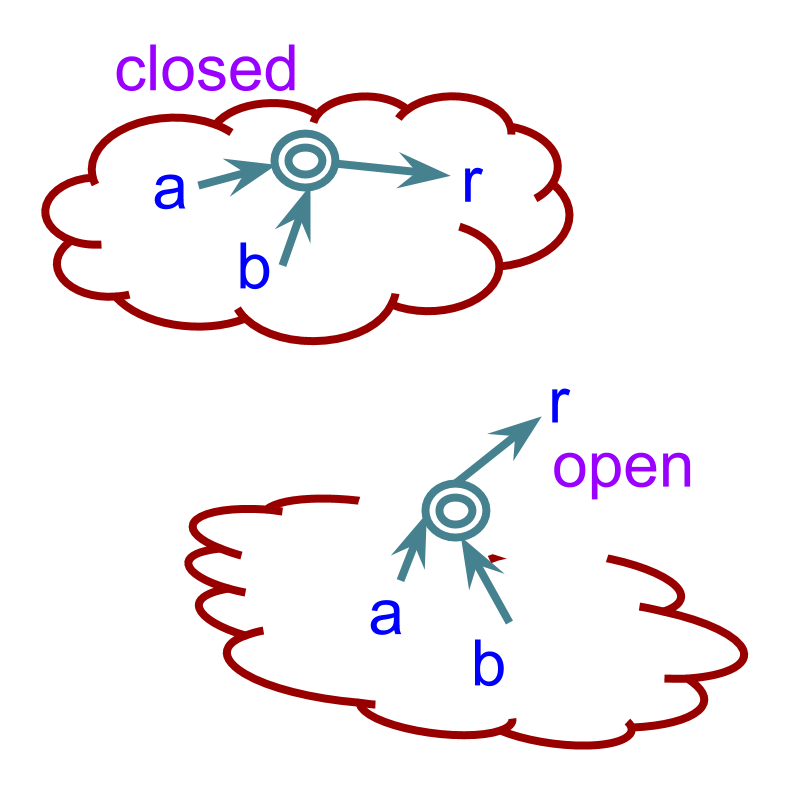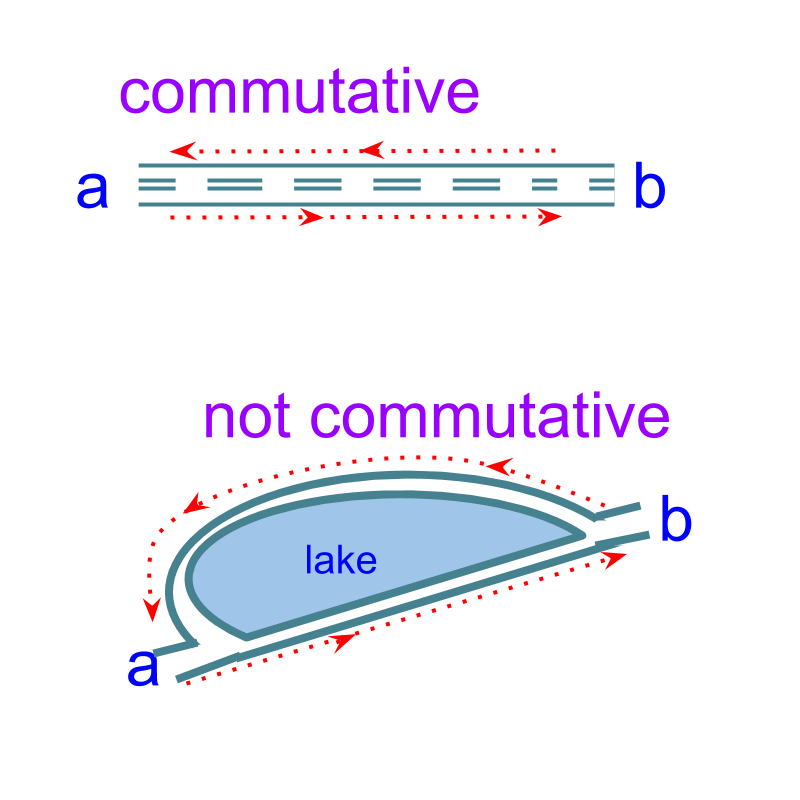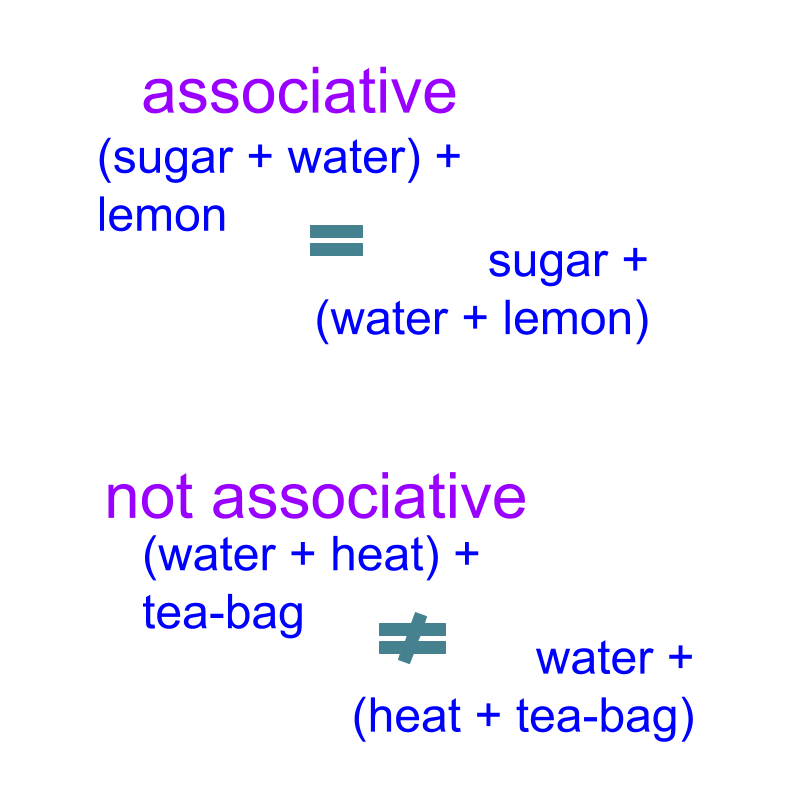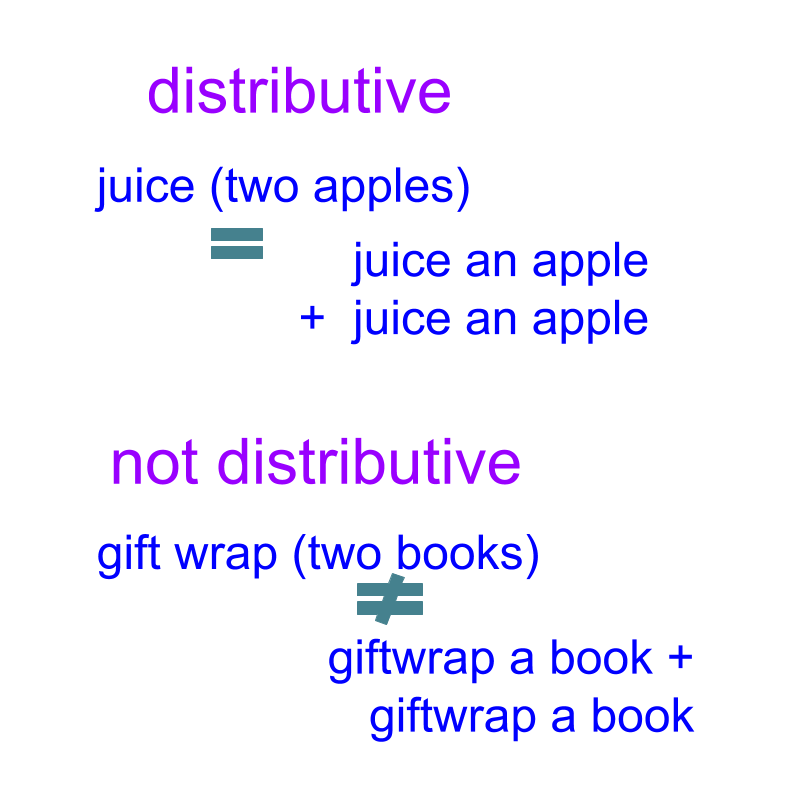maths > algebra

Laws and Properties of Arithmetics : Multiplication

what you'll learn...

Overview

»  Closure Property
→  if $x,y\in ℝ$$x , y \in \mathbb{R}$, then $x×y\in ℝ$$x \times y \in \mathbb{R}$

»  Commutative Property
→  $x×y=y×x$$x \times y = y \times x$

»  Associative Property
→  $\left(x×y\right)×z=x×\left(y×z\right)$$\left(x \times y\right) \times z = x \times \left(y \times z\right)$

→  $\left(x+y\right)×z=x×z+y×z$$\left(x + y\right) \times z = x \times z + y \times z$

»  Multiplicative Identity Property
→  $1\in ℝ$$1 \in \mathbb{R}$, such that $x×1=x$$x \times 1 = x$

→  $\frac{1}{x}\in ℝ$$\frac{1}{x} \in \mathbb{R}$ for any $x\in ℝ$$x \in \mathbb{R}$ (except for $0$$0$) such that $x×\left(\frac{1}{x}\right)=1$$x \times \left(\frac{1}{x}\right) = 1$

»  Division is to be handled as multiplicative inverse for the properties
this is important as algebra extensively involves these properties
→  Commutative property involving division : $x÷y$$x \div y$ is given as $x×\frac{1}{y}=\frac{1}{y}×x$$x \times \frac{1}{y} = \frac{1}{y} \times x$
→  Associative property involving division : $\left(x÷y\right)÷z$$\left(x \div y\right) \div z$ is given as $\left(x×\frac{1}{y}\right)×\frac{1}{z}=x×\left(\frac{1}{y}×\frac{1}{z}\right)$$\left(x \times \frac{1}{y}\right) \times \frac{1}{z} = x \times \left(\frac{1}{y} \times \frac{1}{z}\right)$
→  Distributive property involving division : $p÷q×\left(x+y\right)$$p \div q \times \left(x + y\right)$ is given as $p×\frac{1}{q}×\left(x+y\right)=p×\left(\frac{x}{q}+\frac{y}{q}\right)$$p \times \frac{1}{q} \times \left(x + y\right) = p \times \left(\frac{x}{q} + \frac{y}{q}\right)$

In this lesson, the laws and properties of multiplication is revised. It is very important to go through this once to understand algebra.

closure means within

For any real numbers $p,q\in ℝ$$p , q \in \mathbb{R}$, $p×q$$p \times q$ is a real number.Closure Property of Multiplication: Given $p,q\in ℝ$$p , q \in \mathbb{R}$. $p×q\in ℝ$$p \times q \in \mathbb{R}$.

Closure Property applied to Division: Given $p,q\in ℝ$$p , q \in \mathbb{R}$. $p÷q\in ℝ$$p \div q \in \mathbb{R}$.

Proof:
Given $p,q\in ℝ$$p , q \in \mathbb{R}$
$\frac{1}{q}\in ℝ$$\frac{1}{q} \in \mathbb{R}$ as per Multiplicative Inverse Property
$p×\left(\frac{1}{q}\right)\in ℝ$$p \times \left(\frac{1}{q}\right) \in \mathbb{R}$ as per Closure property of Multiplication
$⇒p÷q\in ℝ$$\implies p \div q \in \mathbb{R}$

Using Closure Property: Given $p,q,r,s\in ℝ$$p , q , r , s \in \mathbb{R}$, $p×q÷r×s$$p \times q \div r \times s$, the subexpression $p×q÷r$$p \times q \div r$ is a number and can be considered as a single number for any other property.

For example as per commutative property $p×q÷r×s=s×p×q÷r$$p \times q \div r \times s = s \times p \times q \div r$, in which $p×q÷r$$p \times q \div r$ is considered to be a single real number.

forward and backward

Given $p,q\in ℝ$$p , q \in \mathbb{R}$. $p×q=q×p$$p \times q = q \times p$Commutative Property of Multiplication: Given $p,q\in ℝ$$p , q \in \mathbb{R}$, $p×q=q×r$$p \times q = q \times r$.

Commutative Property applied to Division: $p÷q=\frac{1}{q}×p$$p \div q = \frac{1}{q} \times p$.
Note: Division has to be handled as inverse of multiplication, $p÷q=p×\left(\frac{1}{q}\right)$$p \div q = p \times \left(\frac{1}{q}\right)$ and then commutative property can be used.

Using Commutative Property: Given $p,q,r\in ℝ$$p , q , r \in \mathbb{R}$, the expression $p×q×p÷q×r÷p×{r}^{2}$$p \times q \times p \div q \times r \div p \times {r}^{2}$ is simplified to $p{r}^{3}$$p {r}^{3}$. students may work this out to understand.

with this or that

Given $p,q,r\in ℝ$$p , q , r \in \mathbb{R}$. $\left(p×q\right)×r=p×\left(q×r\right)$$\left(p \times q\right) \times r = p \times \left(q \times r\right)$Associative Property of Multiplication: Given $p,q,r\in ℝ$$p , q , r \in \mathbb{R}$. $\left(p×q\right)×r=p×\left(q×r\right)$$\left(p \times q\right) \times r = p \times \left(q \times r\right)$.

Associative Property applied to Division:

$\left(p÷q\right)÷r=p×\left(\frac{1}{q}×\frac{1}{r}\right)$$\left(p \div q\right) \div r = p \times \left(\frac{1}{q} \times \frac{1}{r}\right)$.
Note: Division has to be handled as inverse of multiplication, $\left(p÷q\right)÷r=\left(p×\frac{1}{q}\right)×\frac{1}{r}$$\left(p \div q\right) \div r = \left(p \times \frac{1}{q}\right) \times \frac{1}{r}$ and then associative property can be used.

Given $p,q,r\in ℝ$$p , q , r \in \mathbb{R}$. Which of the following equals $\left(p+q\right)×r=p×r+q×r$$\left(p + q\right) \times r = p \times r + q \times r$

The word 'distribute' means 'to share; to spread'Distributive Property of Multiplication: Given $p,q,r\in ℝ$$p , q , r \in \mathbb{R}$. $\left(p+q\right)×r=p×r+q×r$$\left(p + q\right) \times r = p \times r + q \times r$.

Distributive Property applied to Division: There are 3 possible scenarios. In any such scenario, convert the division to inverse of multiplication, and distributive property applies.

(1)  $\left(p+q\right)÷r=\frac{p}{r}+\frac{q}{r}$$\left(p + q\right) \div r = \frac{p}{r} + \frac{q}{r}$.

(2)  $r÷\left(p+q\right)=\frac{r}{p+q}$$r \div \left(p + q\right) = \frac{r}{p + q}$. The multiplication does not distribute in this case.

(3)  $t÷r×\left(p+q\right)=t×\left(\frac{p}{r}+\frac{q}{r}\right)$$t \div r \times \left(p + q\right) = t \times \left(\frac{p}{r} + \frac{q}{r}\right)$.

In algebra, division is always given as $\frac{p}{q}$$\frac{p}{q}$ and not $p÷q$$p \div q$. Thus commutative property, associative property, and distributive property can be used without any unintended errors.

one

Given $p\in ℝ$$p \in \mathbb{R}$. What is $p×1=p$$p \times 1 = p$

Multiplicative Identity Property: For any $p\in ℝ$$p \in \mathbb{R}$, there exists $1\in ℝ$$1 \in \mathbb{R}$ such that $p×1=p$$p \times 1 = p$.

Multiplicative Identity applied to Division: $p÷1=p$$p \div 1 = p$

Note: $\frac{1}{1}=1$$\frac{1}{1} = 1$ and so $p÷1=p×\left(\frac{1}{1}\right)=p×1=p$$p \div 1 = p \times \left(\frac{1}{1}\right) = p \times 1 = p$.

inverse

Given $p\in ℝ$$p \in \mathbb{R}$. What is $p×\frac{1}{p}$$p \times \frac{1}{p}$?

Multiplicative Inverse Property: For any $p\in ℝ$$p \in \mathbb{R}$ and $p\ne 0$$p \ne 0$, there exists $\frac{1}{p}\in ℝ$$\frac{1}{p} \in \mathbb{R}$ such that $p×\frac{1}{p}=1$$p \times \frac{1}{p} = 1$.

summary

The properties together are named as CADI properties of multiplication. The abbreviation CADI is a simplified form of the first letters of Closure, Commutative, Associative, Distributive, Inverse, and Identity properties.

Note: Distributive property is shared with addition.

•  Closure Property
if $x,y\in ℝ$$x , y \in \mathbb{R}$, then $x×y\in ℝ$$x \times y \in \mathbb{R}$

•  Commutative Property
$x×y=y×x$$x \times y = y \times x$

•  Associative Property
$\left(x×y\right)×z=x×\left(y×z\right)$$\left(x \times y\right) \times z = x \times \left(y \times z\right)$

$\left(x+y\right)×z=x×z+y×z$$\left(x + y\right) \times z = x \times z + y \times z$

•  Multiplicative Identity Property
$1\in ℝ$$1 \in \mathbb{R}$, such that $x×1=x$$x \times 1 = x$

•  Multiplicative Inverse Property
$\frac{1}{x}\in ℝ$$\frac{1}{x} \in \mathbb{R}$ for any $x\in ℝ$$x \in \mathbb{R}$ (except for $0$$0$) such that $x×\left(\frac{1}{x}\right)=1$$x \times \left(\frac{1}{x}\right) = 1$

•  Division is to be handled as inverse of multiplication for the properties

This is important as algebra extensively involves these properties.

Commutative property involving division : $x÷y$$x \div y$ is given as $x×\frac{1}{y}=\frac{1}{y}×x$$x \times \frac{1}{y} = \frac{1}{y} \times x$

Associative property involving division : $\left(x÷y\right)÷z$$\left(x \div y\right) \div z$ is given as $\left(x×\frac{1}{y}\right)×\frac{1}{z}=x×\left(\frac{1}{y}×\frac{1}{z}\right)$$\left(x \times \frac{1}{y}\right) \times \frac{1}{z} = x \times \left(\frac{1}{y} \times \frac{1}{z}\right)$

Distributive property involving division : $p÷q×\left(x+y\right)$$p \div q \times \left(x + y\right)$ is given as $p×\frac{1}{q}×\left(x+y\right)=p×\left(\frac{x}{q}+\frac{y}{q}\right)$$p \times \frac{1}{q} \times \left(x + y\right) = p \times \left(\frac{x}{q} + \frac{y}{q}\right)$

Outline

The outline of material to learn "Algebra Foundation" is as follows.

→   Numerical Arithmetics

→   Arithmetic Operations and Precedence

→   Properties of Comparison

→   Properties of Multiplication

→   Properties of Exponents

→   Algebraic Expressions

→   Algebraic Equations

→   Algebraic Identities

→   Algebraic Inequations